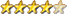Simple Maze using Truchet Tiles

Author Message
William Leue
GuruJoined: 03/07/2020
Location: United States
Posts: 365
 Posted: 08:20pm 05 Apr 2021

I also some bling to mine: the screen now scrolls continuously upward as new rows are added at the bottom, and the color of the swirls now varies slowly and continuously around the wheel of fully saturated bright colors.

-Bill

' Maze formed from Truchet Tiles
' This is basically the same algorithm as the famous
' Commodore Pet '10Print' one-line maze.
' Rev 1.0.0 William M Leue 4/1/2021
option base 1

const TSIZE = 30

mode 1,16
open "debug.txt" for output as #1
cls
ncols = MM.HRES\TSIZE
nrows = MM.VRES\TSIZE
dim float h = 0.0
dim float s = 1.0
dim float v = 1.0
for row = 1 to nrows-1
y = (row-1)*TSIZE
for col = 1 to ncols
x = (col-1)*TSIZE
HSV2RGB(h, s, v, r, g, b)
c = RGB(r, g, b)
DrawTruchetTile x, y, rnd(), c
next col
next row
do
page scroll 0, 0, TSIZE, -1
y = (nrows-2)*TSIZE
for col = 1 to ncols
x = (col-1)*TSIZE
HSV2RGB(h, s, v, r, g, b)
c = RGB(r, g, b)
DrawTruchetTile x, y, rnd(), c
next col
pause 200
h = h + 0.5
if h > 360.0 then h = 0.0
print #1, "HSV2RGB(" + str\$(h) + "," + str\$(s) + "," + str\$(v) + "," + str\$(r) + "," + str\$(g) + "," +  str\$(b)
loop
end

sub DrawTruchetTile x, y, r as float, c
local cx, cy
if r < 0.5 then
cx = x : cy = y + TSIZE
arc cx, cy, TSIZE\2,, 0, 90, c
cx = x + TSIZE : cy = y
arc cx, cy, TSIZE\2,, 180, 270, c
else
cx = x: cy = y
arc cx, cy, TSIZE\2,, 90, 180, c
cx = x + TSIZE: cy = y + TSIZE
arc cx, cy, TSIZE\2,, -90, 0, c
end if
end sub

' ColorConvert.inc -- BASIC include file suitable for inclusion
' wherever color specs using RGB or HSV need to be inter-converted.
' William M Leue 1/7/2021

' Convert an RGB Color to HSV values.
' the RGB values must be in range 0..255; the S and V values will
' be in range 0..1; the H value will be in range 0..360
sub RGB2HSV r, g, b, h as float, s as float, v as float
local float rp, cmax, cmin, delta

rp = r/255.0 : gp = g/255.0 : bp = b/255.0
cmax = max(rp, max(gp, bp))
cmin = min(rp, min(gp, bp))
delta = cmax - cmin
if delta = 0 then
h = 0
else if cmax = rp then
h = 60*(((gp-bp)/delta) MOD 6)
else if cmax = gp then
h = 60*(((bp-rp)/delta) + 2)
else
h = 60*(((rp-gp)/delta) + 4)
end if
if cmax = 0 then
s = 0
else
s = delta/cmax
end if
v = cmax
end sub

' Convert an HSV value to its RGB equivalent
' The S and V values must be in range 0..1; the H value must
' be in range 0..360. The RGB values will be in range 0..255.
sub HSV2RGB h as float, s as float, v as float, r, g, b
local float hh, f, p, q, t, x, c, i
'local i
c = v*s
hh = h/60.0
i = int(hh)
f = hh - i
p = v*(1-s)
q = v*(1-s*f)
t = v*(1-s*(1-f))
x = c*(1.0 - hi MOD 2 - 1)

select case i
case 0
rp = v : gp = t : bp = p
case 1
rp = q : gp = v : bp = p
case 2
rp = p : gp = v : bp = t
case 3
rp = p : gp = q : bp = v
case 4
rp = t : gp = p : bp = v
case 5
rp = v : gp = p : bp = q
end select
r = rp*255 : g = gp*255 : b = bp*255
end sub

' function to return an RGB color, given the h, s, and v
' values as input parameters. The S and V values must be
' in the range 0..1; the H value must be in the range
' 0..360. The output value will be a 24-bit RGB color.
function GetRGBColor(h as float, s as float, v as float)
local r, g, b, c

HSV2RGB h, s, v, r, g, b
c = RGB(r ,g, b)
GetRGBColor = c
end function

' draw color swatches: the left-hand one is the original
' RGB color specified by the user. The right-hand one is
' that color first converted from RGB to HSV, and then
' back again to RGB. The two swatches will be the
' identical color.
sub ShowColors r,g,b,r2,g2,b2
local c1, c2

c1 = RGB(r,g,b)
c2 = RGB(r2,g2,b2)
box 200,200,100,100,1, RGB(WHITE), c1
box 400,200,100,100,1, RGB(WHITE), c2
end sub

sub ShowPixelColors r,g,b
local c1, x, y

box 200,400,100,100,1, RGB(WHITE), c1
c1 = RGB(r,g,b)
for y = 401 to 498
for x = 201 to 298
pixel x, y, c1
next x
next y
end sub